# Cardiac Left Ventricle Segmentation from Cine-MRI Images Using U-Net Network

This example shows how to perform semantic segmentation of the left ventricle from 2-D cardiac MRI images using U-Net.

Semantic segmentation associates each pixel in an image with a class label. Segmentation of cardiac MRI images is useful for detecting abnormalities in heart structure and function. A common challenge of medical image segmentation is class imbalance, meaning the region of interest is small relative to the image background. Therefore, the training images contain many more background pixels than labeled pixels, which can limit classification accuracy. In this example, you address class imbalance by using a generalized Dice loss function . You also use the gradient-weighted class activation mapping (Grad-CAM) deep learning explainability technique to determine which regions of an image are important for the pixel classification decision.

This figure shows an example of a cine-MRI image before segmentation, the network-predicted segmentation map, and the corresponding Grad-CAM map.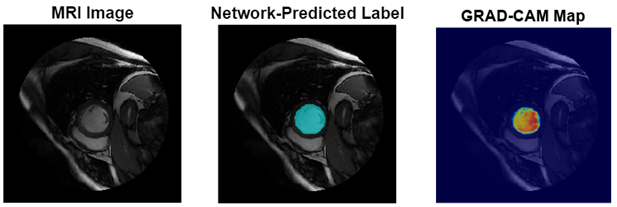Download the pretrained U-Net network by using the `downloadTrainedNetwork` helper function. The helper function is attached to this example as a supporting file. You can use this pretrained network to run the example without training the network.

```exampleDir = fullfile(tempdir,"cardiacMR"); if ~isfolder(exampleDir) mkdir(exampleDir); end trainedNetworkURL = "https://ssd.mathworks.com/supportfiles" + ... "/medical/pretrainedLeftVentricleSegmentation.zip"; downloadTrainedNetwork(trainedNetworkURL,exampleDir);```

```data = load(fullfile(exampleDir,"pretrainedLeftVentricleSegmentationModel.mat")); trainedNet = data.trainedNet;```

### Perform Semantic Segmentation

Use the pretrained network to predict the left ventricle segmentation mask for a test image.

This example uses a subset of the Sunnybrook Cardiac Data data set [2,3]. The subset consists of 45 cine-MRI images and their corresponding ground truth label images. The MRI images were acquired from multiple patients with various cardiac pathologies. The ground truth label images were manually drawn by experts . The MRI images are in the DICOM file format and the label images are in the PNG file format. The total size of the subset of data is ~105 MB.

```zipFile = matlab.internal.examples.downloadSupportFile("medical","CardiacMRI.zip"); filepath = fileparts(zipFile); unzip(zipFile,filepath)```

The `imageDir` folder contains the downloaded and unzipped data set.

`imageDir = fullfile(filepath,"Cardiac MRI");`

Read an image from the data set and preprocess the image by using the `preprocessImage` helper function, which is defined at the end of this example. The helper function resizes MRI images to the input size of the network and converts them from grayscale to three-channel images.

```testImg = dicomread(fullfile(imageDir,"images","SC-HF-I-01","SC-HF-I-01_rawdcm_099.dcm")); trainingSize = [256 256 3]; data = preprocessImage(testImg,trainingSize); testImg = data{1};```

Predict the left ventricle segmentation mask for the test image using the pretrained network by using the `semanticseg` (Computer Vision Toolbox) function.

`segmentedImg = semanticseg(testImg,trainedNet);`

Display the test image and an overlay with the predicted mask as a montage.

```overlayImg = labeloverlay(mat2gray(testImg),segmentedImg, ... Transparency=0.7, ... IncludedLabels="LeftVentricle"); imshowpair(mat2gray(testImg),overlayImg,"montage");```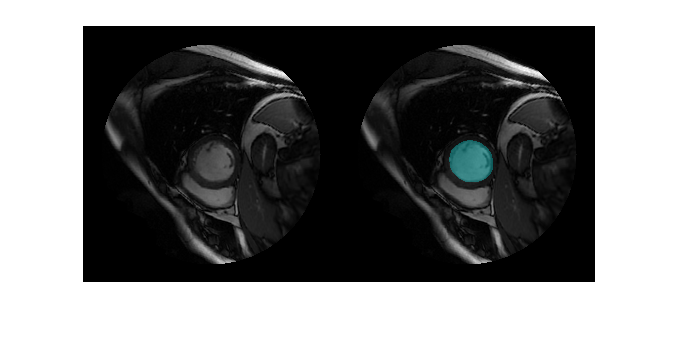### Prepare Data for Training

Create an `imageDatastore` object to read and manage the MRI images.

```dataFolder = fullfile(imageDir,"images"); imds = imageDatastore(dataFolder,... IncludeSubfolders=true,... FileExtensions=".dcm",... ReadFcn=@dicomread);```

Create a `pixelLabelDatastore` (Computer Vision Toolbox) object to read and manage the label images.

```labelFolder = fullfile(imageDir,"labels"); classNames = ["Background","LeftVentricle"]; pixIDs = [0,1]; pxds = pixelLabelDatastore(labelFolder,classNames,pixIDs,... IncludeSubfolders=true,... FileExtensions=".png");```

Preprocess the data by using the `transform` function with custom operations specified by the `preprocessImage` helper function, which is defined at the end of this example. The helper function resizes the MRI images to the input size of the network and converts them from grayscale to three-channel images.

`timds = transform(imds,@(img) preprocessImage(img,trainingSize));`

Preprocess the label images by using the `transform` function with custom operations specified by the `preprocesslabels` helper function, which is defined at the end of this example. The helper function resizes the label images to the input size of the network.

`tpxds = transform(pxds,@(img) preprocessLabels(img,trainingSize));`

Combine the transformed image and pixel label datastores to create a `CombinedDatastore` object.

`combinedDS = combine(timds,tpxds);`

Partition Data for Training, Validation, and Testing

Split the combined datastore into data sets for training, validation, and testing. Allocate 75% of the data for training, 5% for validation, and the remaining 20% for testing.

```numImages = numel(imds.Files); numTrain = round(0.75*numImages); numVal = round(0.05*numImages); numTest = round(0.2*numImages); shuffledIndices = randperm(numImages); dsTrain = subset(combinedDS,shuffledIndices(1:numTrain)); dsVal = subset(combinedDS,shuffledIndices(numTrain+1:numTrain+numVal)); dsTest = subset(combinedDS,shuffledIndices(numTrain+numVal+1:end));```

Visualize the number of images in the training, validation, and testing subsets.

```figure bar([numTrain,numVal,numTest]) title("Partitioned Data Set") xticklabels({"Training Set","Validation Set","Testing Set"}) ylabel("Number of Images")```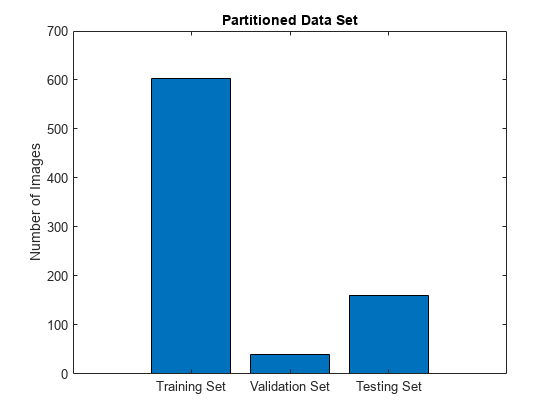Augment Training Data

Augment the training data by using the `transform` function with custom operations specified by the `augmentDataForLVSegmentation` helper function, which is defined at the end of this example. The helper function applies random rotations, translations, and reflections to the MRI images and corresponding ground truth labels.

`dsTrain = transform(dsTrain,@(data) augmentDataForLVSegmentation(data));`

Measure Label Imbalance

To measure the distribution of class labels in the data set, use the `countEachLabel` function to count the background pixels and the labeled ventricle pixels.

`pixelLabelCount = countEachLabel(pxds)`
```pixelLabelCount=2×3 table Name PixelCount ImagePixelCount _________________ __________ _______________ {'Background' } 5.1901e+07 5.2756e+07 {'LeftVentricle'} 8.5594e+05 5.2756e+07 ```

Visualize the labels by class. The image contains many more background pixels than labeled ventricle pixels. The label imbalance can bias the training of the network. You address this imbalance when you design the network.

```figure bar(categorical(pixelLabelCount.Name),pixelLabelCount.PixelCount) ylabel("Frequency")```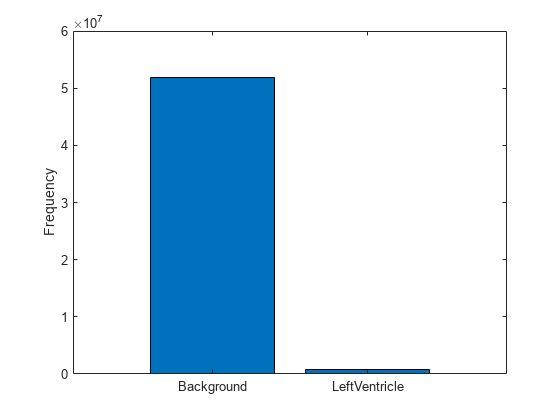### Define Network Architecture

This example uses a U-Net network for semantic segmentation. Create a U-Net network with an input size of 256-by-256-by-3 that classifies pixels into two categories corresponding to the background and left ventricle.

```numClasses = length(classNames); net = unetLayers(trainingSize,numClasses);```

Replace the input network layer with an `imageInputLayer` (Deep Learning Toolbox) object that normalizes image values between 0 and 1000 to the range [0, 1]. Values less than 0 are set to 0 and values greater than 1000 are set to 1000.

```inputlayer = imageInputLayer(trainingSize, ... Normalization="rescale-zero-one", ... Min=0, ... Max=1000, ... Name="input"); net = replaceLayer(net,net.Layers(1).Name,inputlayer);```

To address the class imbalance between the smaller ventricle regions and larger background, this example uses a `dicePixelClassificationLayer` (Computer Vision Toolbox) object. Replace the pixel classification layer with the Dice pixel classification layer.

```pxLayer = dicePixelClassificationLayer(Name="labels"); net = replaceLayer(net,net.Layers(end).Name,pxLayer);```

### Specify Training Options

Specify the training options by using the `trainingOptions` (Deep Learning Toolbox) function. Train the network using the `adam` optimization solver. Set the learning rate to 0.001 over the span of training. You can experiment with the mini-batch size based on your GPU memory. Batch normalization layers are less effective for smaller values of the mini-batch size. Tune the initial learning rate based on the mini-batch size.

```options = trainingOptions("adam", ... InitialLearnRate=0.0002,... GradientDecayFactor=0.999,... L2Regularization=0.0005, ... MaxEpochs=100, ... MiniBatchSize=32, ... Shuffle="every-epoch", ... Verbose=false,... VerboseFrequency=100,... ValidationData=dsVal,... Plots="training-progress",... ExecutionEnvironment="auto",... ResetInputNormalization=false);```

### Train Network

To train the network, set the `doTraining` variable to `true`. Train the network by using the `trainNetwork` (Deep Learning Toolbox) function.

Train on a GPU if one is available. Using a GPU requires a Parallel Computing Toolbox™ license and a CUDA®-enabled NVIDIA® GPU.

```doTraining =false; if doTraining trainedNet = trainNetwork(dsTrain,net,options); modelDateTime = string(datetime("now",Format="yyyy-MM-dd-HH-mm-ss")); save(fullfile(exampleDir,"trainedLeftVentricleSegmentation-" ... +modelDateTime+".mat"),"trainedNet"); end```

### Test Network

Segment each image in the test data set by using the trained network.

```resultsDir = fullfile(exampleDir,"Results"); if ~isfolder(resultsDir) mkdir(resultsDir) end pxdsResults = semanticseg(dsTest,trainedNet,... WriteLocation=resultsDir,... Verbose=true,... MiniBatchSize=1);```
```Running semantic segmentation network ------------------------------------- * Processed 161 images. ```

Evaluate Segmentation Metrics

Evaluate the network by calculating performance metrics using the `evaluateSemanticSegmentation` (Computer Vision Toolbox) function. The function computes metrics that compare the labels that the network predicts in `pxdsResults` to the ground truth labels in `pxdsTest`.

```pxdsTest = dsTest.UnderlyingDatastores{2}; metrics = evaluateSemanticSegmentation(pxdsResults,pxdsTest);```
```Evaluating semantic segmentation results ---------------------------------------- * Selected metrics: global accuracy, class accuracy, IoU, weighted IoU, BF score. * Processed 161 images. * Finalizing... Done. * Data set metrics: GlobalAccuracy MeanAccuracy MeanIoU WeightedIoU MeanBFScore ______________ ____________ _______ ___________ ___________ 0.99829 0.97752 0.9539 0.99667 0.96747 ```

View the metrics by class by querying the `ClassMetrics` property of `metrics`.

`metrics.ClassMetrics`
```ans=2×3 table Accuracy IoU MeanBFScore ________ _______ ___________ Background 0.99907 0.99826 0.995 LeftVentricle 0.95598 0.90953 0.93994 ```

Evaluate Dice Score

Evaluate the segmentation accuracy by calculating the Dice score between the predicted and ground truth label images. For each test image, calculate the Dice score for the background label and the ventricle label by using the `dice` function.

```reset(pxdsTest); reset(pxdsResults); diceScore = zeros(numTest,numClasses); for idx = 1:numTest prediction = read(pxdsResults); groundTruth = read(pxdsTest); diceScore(idx,1) = dice(prediction{1}==classNames(1),groundTruth{1}==classNames(1)); diceScore(idx,2) = dice(prediction{1}==classNames(2),groundTruth{1}==classNames(2)); end```

Calculate the mean Dice score over all test images and report the mean values in a table.

```meanDiceScore = mean(diceScore); diceTable = array2table(meanDiceScore', ... VariableNames="Mean Dice Score", ... RowNames=classNames)```
```diceTable=2×1 table Mean Dice Score _______________ Background 0.99913 LeftVentricle 0.9282 ```

Visualize the Dice scores for each class as a box chart. The middle blue line in the plot shows the median Dice score. The upper and lower bounds of the blue box indicate the 25th and 75th percentiles, respectively. Black whiskers extend to the most extreme data points that are not outliers.

```figure boxchart(diceScore) title("Test Set Dice Accuracy") xticklabels(classNames) ylabel("Dice Coefficient")```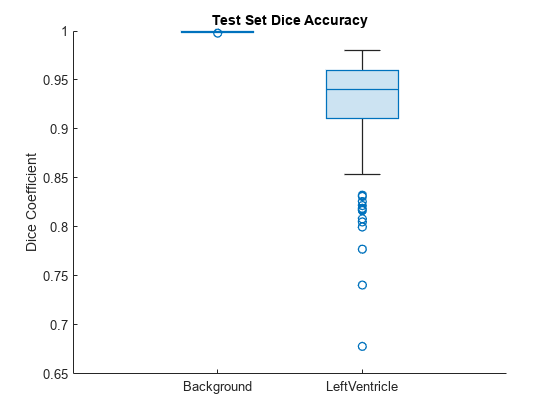### Explainability

By using explainability methods like Grad-CAM, you can see which areas of an input image the network uses to make its pixel classifications. Use Grad-CAM to show which areas of a test MRI image the network uses to segment the left ventricle.

Load an image from the test data set and preprocess it using the same operations you use to preprocess the training data. The `preprocessImage` helper function is defined at the end of this example.

```testImg = dicomread(fullfile(imageDir,"images","SC-HF-I-01","SC-HF-I-01_rawdcm_099.dcm")); data = preprocessImage(testImg,trainingSize); testImg = data{1};```

Load the corresponding ground truth label image and preprocess it using the same operations you use to preprocess the training data. The `preprocessLabels` function is defined at the end of this example.

```testGroundTruth = imread(fullfile(imageDir,"labels","SC-HF-I-01","SC-HF-I-01gtmask0099.png")); data = preprocessLabels({testGroundTruth}, trainingSize); testGroundTruth = data{1};```

Segment the test image using the trained network.

`prediction = semanticseg(testImg,trainedNet);`

To use Grad-CAM, you must select a feature layer from which to extract the feature map and a reduction layer from which to extract the output activations. Use `analyzeNetwork` (Deep Learning Toolbox) to find the layers to use with Grad-CAM. In this example, you use the final ReLU layer as the feature layer and the softmax layer as the reduction layer.

```analyzeNetwork(trainedNet) featureLayer = "Decoder-Stage-4-Conv-2"; reductionLayer = "Softmax-Layer";```

Compute the Grad-CAM map for the test image by using the `gradCAM` (Deep Learning Toolbox) function.

```gradCAMMap = gradCAM(trainedNet,testImg,classNames,... ReductionLayer=reductionLayer,... FeatureLayer=featureLayer);```

Visualize the test image, the ground truth labels, the network-predicted labels, and the Grad-CAM map for the ventricle. As expected, the area within the ground truth ventricle mask contributes most strongly to the network prediction of the ventricle label.

```figure tiledlayout(2,2) nexttile imshow(mat2gray(testImg)) title("Test Image") nexttile imshow(labeloverlay(mat2gray(testImg),testGroundTruth)) title("Ground Truth Label") nexttile imshow(labeloverlay(mat2gray(testImg),prediction,IncludedLabels="LeftVentricle")) title("Network-Predicted Label") nexttile imshow(mat2gray(testImg)) hold on imagesc(gradCAMMap(:,:,2),AlphaData=0.5) title("GRAD-CAM Map") colormap jet```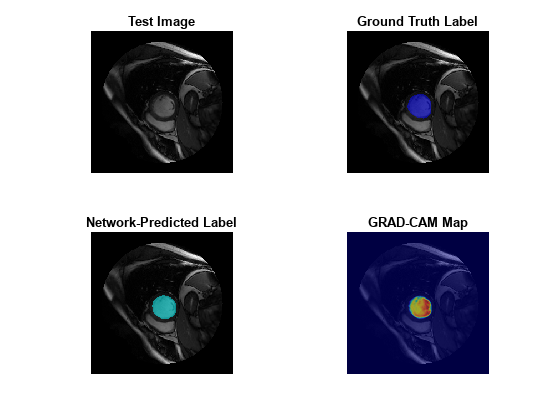### Supporting Functions

The `preprocessImage` helper function preprocesses the MRI images using these steps:

1. Resize the input image to the target size of the network.

2. Convert grayscale images to three channel images.

3. Return the preprocessed image in a cell array.

```function out = preprocessImage(img,targetSize) % Copyright 2023 The MathWorks, Inc. targetSize = targetSize(1:2); img = imresize(img,targetSize); if size(img,3) == 1 img = repmat(img,[1 1 3]); end out = {img}; end```

The `preprocessLabels` helper function preprocesses label images using these steps:

1. Resize the input label image to the target size of the network. The function uses nearest neighbor interpolation so that the output is a binary image without partial decimal values.

2. Return the preprocessed image in a cell array.

```function out = preprocessLabels(labels, targetSize) % Copyright 2023 The MathWorks, Inc. targetSize = targetSize(1:2); labels = imresize(labels{1},targetSize,"nearest"); out = {labels}; end```

The `augmentDataForLVSegmentation` helper function randomly applies these augmentations to each input image and its corresponding label image. The function returns the output data in a cell array.

• Random rotation between 0 to 180 degrees.

• Random translation along the x- and y-axes of -10 to 10 pixels.

• Random reflection to flip the image in the x-axis.

```function out = augmentDataForLVSegmentation(data) % Copyright 2023 The MathWorks, Inc. img = data{1}; labels = data{2}; inputSize = size(img,[1 2]); tform = randomAffine2d(... Rotation=[-5 5],... XTranslation=[-10 10],... YTranslation=[-10 10]); sameAsInput = affineOutputView(inputSize,tform,BoundsStyle="sameAsInput"); img = imwarp(img,tform,"linear",OutputView=sameAsInput); labels = imwarp(labels,tform,"nearest",OutputView=sameAsInput); out = {img,labels}; end```

### References

 Milletari, Fausto, Nassir Navab, and Seyed-Ahmad Ahmadi. “V-Net: Fully Convolutional Neural Networks for Volumetric Medical Image Segmentation.” In 2016 Fourth International Conference on 3D Vision (3DV), 565–71. Stanford, CA, USA: IEEE, 2016. https://doi.org/10.1109/3DV.2016.79.

 Radau, Perry, Yingli Lu, Kim Connelly, Gideon Paul, Alexander J Dick, and Graham A Wright. “Evaluation Framework for Algorithms Segmenting Short Axis Cardiac MRI.” The MIDAS Journal, July 9, 2009. https://doi.org/10.54294/g80ruo.

 “Sunnybrook Cardiac Data – Cardiac Atlas Project.” Accessed January 10, 2023. http://www.cardiacatlas.org/studies/sunnybrook-cardiac-data/.